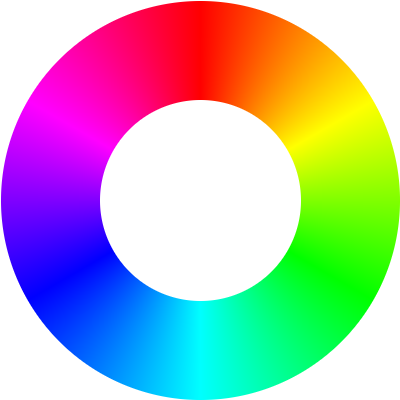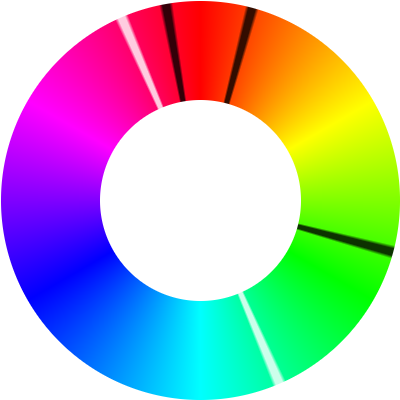# How to average hues

On ·

Recently I needed some software to be able to average the hues in some HSL colors to find the hue between them. My first attempt was simple: just treat the hues as a number from 0° to 360° and average them. That approach did not work as I expected. The solution was to take a circular mean; this post will explain that.

Here’s what a naive arithmetic mean would look like for averaging 40° and 300°. The average of two numbers is always equidistant between those two numbers, so the average sits right in the middle of the two averaged numbers:That averaged color doesn’t look right. We averaged orange and magenta but got a greenish cyan. Something like red would have been a better in-between color.

## The color wheel

The issue with our averaging scheme is that it treats colors on the left and right side as being far apart, when in reality the color line loops around. It’s really a wheel:## A simple way to average 2 hues

There’s a simple way to find a good average of 2 hues, but it doesn’t work for more than two:

Expand

On a wheel, there are two equidistant points from the two averaged numbers, shown in white. The one on the top of the circle is closer to the two inputs, and the other is further from the two inputs. Here, the two averaged numbers are plotted in black, and the averages in white:

The calculation for the two averages is simple: we can find one the usual way, by taking the average of the hues:

function simpleAvg(p1, p2) {
return (p1 + p2) / 2 }


And rotate the first average by 180° to get the second one:

function oppositeAvg(p1, p2) {
return (simpleAvg(p1, p2) + 180) % 360 }


We can use the average closest to the points as our average hue.

## More than 2 hues

Here’s a color wheel with three values (in black) to average:The two values in white are derived from the arithmetic average of all three hues. None of them look right.

We can take a circular mean to find a hue that does look right. Here’s the process to find one (click it to advance the current step):

1. Make each hue a point on the circle
Each angle is converted into a point on a circle.
2. Find the average point
The X and Y values of the points on the circle are averaged to find an average point.
3. Find the angle of the average point
The angle of the average point is the circular average.

By treating the colors as 2D points and finding the point between them all on the color wheel, we get a much better average.

## Calculating the circular average

Here’s how we could implement that in JavaScript (all angles are in radians since that’s what the JS trigonometric functions use):

function hueAverage(hues) {
// Convert each hue to a point on the unit circle
const points = hues.map(hue => [Math.cos(hue), Math.sin(hue)]);

// Find the average point
const averagePoint = [
points.reduce((prev, cur) => prev + cur, 0) / points.length, // x
points.reduce((prev, cur) => prev + cur, 0) / points.length, // y
];

// Get the angle of the average point
return Math.atan2(averagePoint, averagePoint);
}

hueAverage([Math.PI/6, 2*Math.PI - Math.PI/6]) // -> almost 0


hueAverage can be optimized a bit by seperating the calculation of the x and y averages.

Here’s a mathematical formula that finds the circular average:

$\text{avg}\left(l\right)=\text{atan2}\left(\frac{\sum _{i}\text{sin}\left({l}_{i}\right)}{\text{len}\left(l\right)},\frac{\sum _{i}\text{cos}\left({l}_{i}\right)}{\text{len}\left(l\right)}\right)$

Math.atan2 is a cool function. It takes a (x,y) point, and tells you what angle around the unit circle the point is. Here’s a nice illustration:Keep in mind that the arguments are opposite the usual order, since atan2 is a generalization of atan(y/x) that works when x or y is negative.